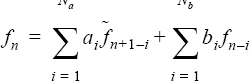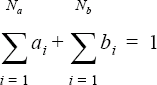# EXTERNAL_CODE

Specifies communication parameters for coupling with an external solid/structural code.

AcuSolve Command

## Syntax

EXTERNAL_CODE {parameters}

## Qualifier

This command has no qualifier.

## Parameters

communication (enumerated) [=socket]
Method of communication between AcuSolve and the external code.
pipe
Pipe communication. External code reads AcuSolve messages from standard in and writes to standard out. Requires launch_command.
socket
Socket connection on a given port. Requires socket_initiate and socket_port.
fifo
Use a fifo special file. Requires fifo_send_file and fifo_receive_file.
communication (string) [no default]
The command, with parameters, that executes the external code. Used with pipe communication.
socket_initiate (boolean) [=on]
Flag specifying AcuSolve to initiate the socket connection. Otherwise the external code will post the socket. Used with socket communication.
socket_host or host (string) [no default]
User-given name of the host that runs the external code. Used with socket communication and socket_initiate = off.
socket_port or port (integer) >=1 (=10000)
Socket port for communication with external code. Used with socket communication.
fifo_send_file (string) [no default]
User-given name of the fifo special file that the external code opens for reading. Used with fifo communication.
User-given name of the fifo special file that the external code opens for writing. Used with fifo communication.
multiplier_function (string) [=none]
User-given name of the multiplier function for scaling the computed forces, moments, and heat fluxes from AcuSolve before sending them to the external code. If none, no scaling is performed.
filter (enumerated) [=none]
Method of filtering the computed forces, moments and heat fluxes from AcuSolve before sending them to the external code.
none
No filter.
iir
Infinite impulse response digital filter. Requires iir_input_coefficients and iir_output_coefficients.
iir_input_coefficients (array) [={1}]
Infinite impulse response digital filter coefficients applied to computed values. Used with iir filter.
iir_output_coefficients (array) [={}]
Infinite impulse response digital filter coefficients applied to previously filtered values. Used with iir filter.

## Description

This command provides support for solving Direct Coupling Fluid Structure Interaction (DC-FSI) problems by allowing AcuSolve and an external solid/structural code to run in a coupled manner. Forces, moments, and heat fluxes are communicated to the solid/structural code, and displacements are returned to AcuSolve. The code coupling interface (CCI) library handles the required communication and interpolations. Once a solid/structural code is linked to this library, that code can use CCI functions to run in conjunction with AcuSolve to solve a DC-FSI problem. Both explicit and implict couplings with the external code are possible. See also the EQUATION, TIME_SEQUENCE, and EXTERNAL_CODE_SURFACE commands. Complete documentation for CCI is in the file doc/code_coupling_interface.txt, which can be found in the AcuSolve distribution.

The communication parameter determines which of three protocols is used. For example,
EXTERNAL_CODE {
communication       = pipe
launch_command      = "program args"
}

Here AcuSolve launches (only from processor 1) the external code (and arguments) as given by the launch_command parameter. This program must read AcuSolve messages from standard in and write to standard out.

An example of the socket protocol is given by:
EXTERNAL_CODE {
communication       = socket
socket_initiate     = off
socket_host         = "hostname"
socket_port         = 10000
}

If socket_initiate is on, AcuSolve will initiate the socket connection on port socket_port. It is then the responsibility of the external code to connect to that socket. Following this, all communications will be done through sockets. Alternatively, the external code may post the socket when socket_initiate=off. In this case, AcuSolve (from processor 1) connects to the posted socket on the machine given by socket_host and port given by socket_port.

The third protocol is fifo:
EXTERNAL_CODE {
communication             = fifo
fifo_send_file            = "acuSolve2externalCode.txt"
}

This is a quick way of implementing communication between two codes. The external code must open fifo_send_file for reading and fifo_receive_file for writing. Processor one of AcuSolve and the external code need to be on the same Linux or Unix machine. Windows is not supported. The fifo files are created by using the mkfifo Unix utility.

The accuracy and stability of the solution of the DC-FSI system depend on how the external code equations are coupled to the fluid equations. Two strategies are supported:
• Conventional Sequential Staggered (CSS): This uses a single-pass of displacements/rotations/temperatures and forces/moments/heat-fluxes at each time step. The result is essentially an explicit scheme since the data comes from the previous time step. CSS is used when there is exactly one nonlinear stagger per time step (max_stagger_iterations=1 in the STAGGER command).
• Multi-Iterative Coupling (MIC): This strategy corrects the interfacial forces via a multi-pass transfer of displacements/rotations/temperatures and fluid forces/moments/heat-fluxes. From numerical experiments, robustness and efficiency of the scheme are obtained for a mass-density and specific heat ratios of O(1). The scheme requires at least two nonlinear stagger iterations per time step. The interfacial force/moment/heat flux and displacement/rotation/temperature residuals can be obtained by printing the *.Log file, generated by AcuRun, with a verbose level of two.
There are two ways to modify the fluid forces, moments, and heat fluxes before they are sent to the solid/structural code. Such a modification is often necessary to get a robust solution, especially at start up. The first is by specifying a multiplier function:
EXTERNAL_CODE {
communication                     = pipe
launch_command                    = "program args"
multiplier_function               = "ramp up"
}
MULTIPLIER_FUNCTION( "ramp up" ) {
type                                = piecewise_linear
curve_fit_values                    = {  0., 0. ;
10., 1. ;
100., 1. ; }

curve_fit_variable                     = time
}

This example has the effect of decoupling the two codes initially and then linearly ramping up the forces over the first 10 seconds until the full fluid forces are applied within the external code. A multiplier function of mic filter type can also be used. This is most effective when there at least two nonlinear stagger iterations per time step.

The second method uses a digital filter to smooth the forces in time. An iir filter gives an infinite impulse response filter, which is defined by:(1)with the constraint that(2)where $a{}_{i},\text{\hspace{0.17em}}i=1,...,{N}_{a}$ is given by iir_input_coefficients; $b{}_{i},\text{\hspace{0.17em}}i=1,...,{N}_{b}$ is given by iir_output_coefficients; fn is a component of the fluid force applied to the structure at time step n; and ${\stackrel{˜}{f}}_{n}$ is the value of this component before filtering at time step n. ${N}_{a}$ 0 and ${N}_{b}$ are determined by the number of elements of the corresponding arrays. If the constraint is not satisfied, AcuSolve automatically scales the coefficients appropriately. For example,

EXTERNAL_CODE {
...
filter                       = iir
iir_input_coefficients       = { 0.8, 0.8 }
iir_output_coefficients      = { -0.6 }
}